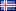ÍslenskaEnglish

27.681
4.598
1.524
2.318

# Lokaverkefni (Meistara)

Vinsamlegast notið þetta auðkenni þegar þið vitnið til verksins eða tengið í það: http://hdl.handle.net/1946/6224

Titill:
• The Pricing of Options with Jump Diffusion and Stochastic Volatility
Námsstig:
• Meistara
Höfundur:
Leiðbeinandi:
Efnisorð:
Útdráttur:
• The Black-Scholes model has been widely used in option pricing for roughly four decades. However, there are two puzzles that have turned out to be difficult to explain with the Black-Scholes model: the leptokurtic feature and the volatility smile. In this study, the two puzzles will be investigated. An improved model – a double exponential jump diffusion model (referred to as the Kou model) will be introduced. The Monte Carlo method will be used to simulate the Black-Scholes model and the double exponential jump diffusion model to price the IBM call option. The call option prices estimated by both models will be compared to the market call prices. The results show that the call option prices estimated by the double exponential jump diffusion model fit the IBM market call option prices better than the call option prices estimated by the Black-Scholes model do. The volatility in both the Black-Scholes model and the double exponential jump diffusion model is assumed to be a constant. However, it is stochastic in reality. Two new models based on the Black-Scholes model and the double exponential jump diffusion model with a stochastic volatility will be developed. The stochastic volatility is determined by a GARCH(1,1) model. The advantage of GARCH model is that GARCH model captures some features associated with financial time series, such as, fat tails, volatility clustering, and leverage effects. First, the structure ofboth the new models will be presented. Then the Monte Carlo simulation will be applied to the two new models to price the IBM call option. Finally, the call option prices estimated by the four models will be compared to the market call option prices. The performance of the four models will be evaluated by statistical methods such as mean absolute error (MAE), mean square error (MSE), root mean square error (RMSE), normalized root mean square error (NRMSE), and information ratio (IR). The results show that the double exponential jump diffusion model performs the best for the lower strike prices and the Kou & GARCH model has the best performance for the higher strike prices. Theoretically, the Kou & GARCH model is expected to be the best model among the four models. Some possible reasons and suggestions will be given in the discussion.

Samþykkt:
• 14.9.2010
URI:
• http://hdl.handle.net/1946/6224

Skrár
Skráarnafn Stærð AðgangurLýsingSkráartegund
Linghao_Yi.pdf1.5 MBOpinnHeildartextiPDF